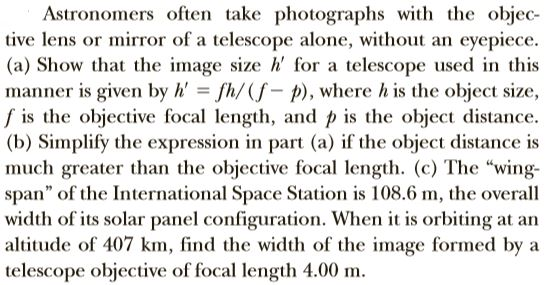# Astronomers often take photographs with the objec-tive lens or mirror of a telescope alone, without an eyepiece.(a) Show that the image size h' for a telescope used in thismanner is given by h' fh/(f- p), where h is the object size,f is the objective focal length, and p is the object distance.(b) Simplify the expression in part (a) if the object distance ismuch greater than the objective focal length. (c) The "wing-span" of the International Space Station is 108.6 m, the overallwidth of its solar panel configuration. When it is orbiting at analtitude of 407 km, find the width of the image formed by atelescope objective of focal length 4.00 m.

Question
1 viewshelp_outlineImage TranscriptioncloseAstronomers often take photographs with the objec- tive lens or mirror of a telescope alone, without an eyepiece. (a) Show that the image size h' for a telescope used in this manner is given by h' fh/(f- p), where h is the object size, f is the objective focal length, and p is the object distance. (b) Simplify the expression in part (a) if the object distance is much greater than the objective focal length. (c) The "wing- span" of the International Space Station is 108.6 m, the overall width of its solar panel configuration. When it is orbiting at an altitude of 407 km, find the width of the image formed by a telescope objective of focal length 4.00 m. fullscreen
check_circle

Step 1

Formula to calculate objective focal length is,

Step 2

Formula to calculate magnification factor is

Step 3

b). The expression of image height...

### Want to see the full answer?

See Solution

#### Want to see this answer and more?

Solutions are written by subject experts who are available 24/7. Questions are typically answered within 1 hour.*

See Solution
*Response times may vary by subject and question.
Tagged in
SciencePhysics

### Ray Optics